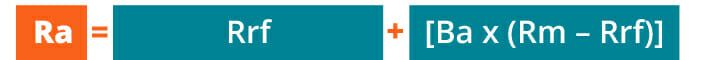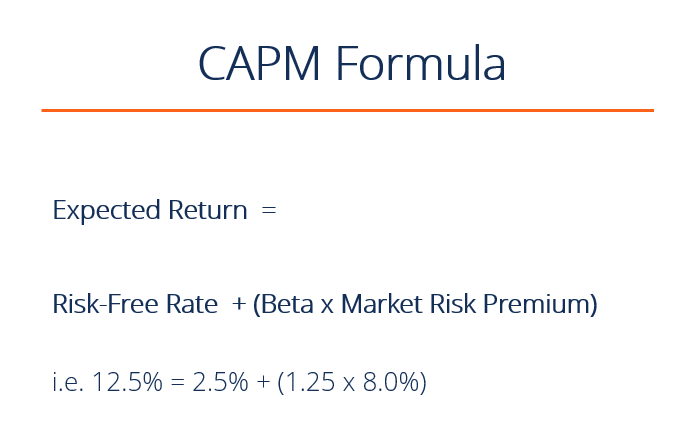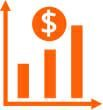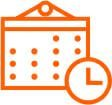# Capital Asset Pricing Model (CAPM)

A method for calculating the required rate of return, discount rate or cost of capital

## What is CAPM?

The Capital Asset Pricing Model (CAPM) is a model that describes the relationship between expected return and risk of investing in a security. It shows that the expected return on a security is equal to the risk-free return plus a risk premium, which is based on the beta of that security. Below is an illustration of the CAPM concept.### CAPM formula and calculation

CAPM is calculated according to the following formula:

#####Where:

Ra = Expected return on a security
Rrf = Risk-free rate
Ba = Beta of the security
Rm = Expected return on market

Note: “Risk Premium” = (Rm – Rrf)

The CAPM formula is used to calculate the expected return on investable asset. It is based on the premise that investors have assumptions of systematic risk (also known as market risk or non-diversifiable risk) and need to be compensated for it in the form of a risk premium – an amount of market return greater than the risk-free rate. By investing in a security, investors want a higher return for taking on additional risk.### Expected returnThe “Ra” notation above represents the expected return of a capital asset over time, given all of the other variables in the equation.  The expected return is a long-term assumption about how an investment will play out over its entire life.

### Risk-free rateThe “Rff” notation is for the risk-free rate, which is typically equal to the yield on a 10-year US government bond.  The risk-free rate should correspond to the country where the investment is being made, and the maturity of the bond should match the time horizon of the investment. Professional convention, however, is to typically use the 10-year rate no matter what, because it’s the most heavily quoted and most liquid bond.

To learn more, check out our free fixed-income fundamentals course.

### BetaThe beta (denoted as “Ba” in the CAPM formula) is a measure of a stock’s risk (volatility of returns) reflected by measuring the fluctuation of its price changes relative to the overall market. In other words, it is the stock’s sensitivity to market risk. For instance, if a company’s beta is equal to 1.5, the security has 150% of the volatility of the market average. However, if the beta is equal to 1, the expected return on a security is equal to the average market return.  A beta of -1 means the security has a perfect negative correlation with the market.

### Market risk premium

From the above components of CAPM we can simplify the formula to reduce (expected return of the market – risk free rate) to be the “market risk premium”.  The market risk premium is the excess return expected to compensate an investor for the additional volatility and risk they will experience over and above the risk-free rate.

### Why CAPM is important

The CAPM formula is widely used in the finance industry by various professions such as investment bankers, financial analysts, and accountants. It is an integral part of the weighted average cost of capital (WACC), as CAPM calculates the cost of equity.

WACC is used extensively in financial modeling.  It can be used to find the net present value (NPV) of the future cash flows of an investment and to further calculate its enterprise value and finally its equity value.

### CAPM example – Calculation of expected return

Let’s calculate the expected return on a stock, using the Capital Asset Pricing Model (CAPM) formula. Suppose the following information about a stock is known:

• It trades on the NYSE and its operations are based in the United States
• The current yield on a U.S. 10-year treasury is 2.5%
• The average excess historical annual return for U.S. stocks is 7.5%
• The beta of the stock is 1.25 (meaning it’s average weekly return is 1.25x as volatile as the S&P500 over the last 2 years)

What is the expected return of the security using the CAPM formula?

Let’s break down the answer using the formula from above in the article:

• Expected return = Risk Free Rate + [Beta x Market Return Premium]
• Expected return = 2.5% + [1.25 x 7.5%]
• Expected return = 11.9%

Enter your name and email in the form below and download the free template now!

### More Resources

We hope this guide to the CAPM formula has been helpful.  To continue learning and advancing your finance career as a financial analyst, we have developed several more articles that are highly relevant.  To learn more please see:

• WACC
• Asset beta
• Valuation methods
• Comparable company analysis
• Financial modeling guide

### Financial Analyst Training

Get world-class financial training with CFI’s online certified financial analyst training program!

Gain the confidence you need to move up the ladder in a high powered corporate finance career path.

Learn financial modeling and valuation in Excel the easy way, with step-by-step training.